# Dark Matter for the Young Physicist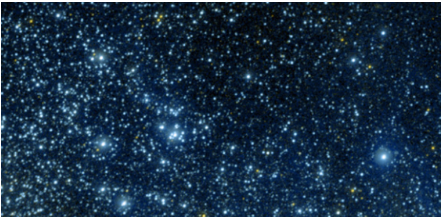Visible stars are not the only matter in space. Credit: (NASA/JPL)

In many, if not most high school physics curriculums, it is common to work extremely hard for a whole year and still be clueless about any of the physics that scientists have learned since the end of the 19th century. Many people who have taken a physics class will never be introduced to the questions of contemporary physics unless they take extensive college coursework. Read on if you do not want to wait till college to learn about a 21st century problem.

The nature and distribution of matter in our universe is one of the main open issues in contemporary physics. When talking about what’s out there, one useful classification is “luminous matter” vs. “dark matter”. Luminous matter is stuff that emits light. This is primarily stars, glowing gas in nebulae, etc. Dark matter is matter that doesn’t glow--hence the moniker dark matter.

It is pretty easy to map the amount and distribution of luminous matter in the universe (just look up into the night sky and start counting stars, for starters). It is much harder to figure out where the dark matter is. Some telescopes and satellites map the distribution of dark matter by examining how it affects the light we see from luminous matter. Other telescopes search for signals as to what exactly dark matter might be made of. Particle accelerators and other experiments also attempt to create and measure dark matter on earth. All this cutting edge science, involving thousands of physicists and scientists every day; started with a simple observation about how stars in galaxies orbit in the context of Newton’s law of gravitation.

The moon orbits the earth, the earth orbits the sun, and the sun orbits...what? Just like satellites and planets, stars can have orbits too. In general, stars in spiral galaxies like the Milky Way orbit around the center of the galaxy they belong to. If a star has a mass mstar and orbits around the center of a galaxy; then the center of a galaxy better have a mass much greater than mstar. Our own sun has a mass much greater mass than the earth. Spiral galaxies do in fact have a large bulge of mass in their centers around which the stars in a galaxy orbit.

An orbit has a radius associated with it, and so we need a good unit of distance to talk about this radius. We measure distances within galaxies in light-years. One light year is approximately 1016 m. As seen below, the radius of the Sun’s orbit around the center of the Milky Way is 28,000 light years.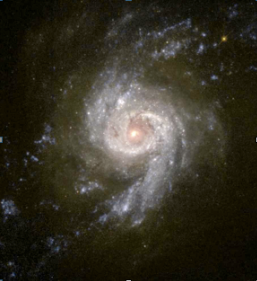Spiral galaxy from above Spiral Galaxy as seen from above. Credit: (NASA) follow link for more cool pictures of spiral galaxies.

Even though the radius of a typical star’s orbit around a galactic bulge is vastly larger than the distances in the solar system, Newton’s laws of gravity still apply to a star’s orbit. In particular, the magnitude of the gravitational force F on the star is given by: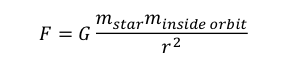Gravitational force on star

where G is Newton’s gravitational constant, $6.67 \times 10-11 N m^2/kg^2, m_{star}$ is the mass of the star, minside orbit is the mass inside the star’s orbit, and r is the radius of the orbit.

Now, one may immediately ask, “Wait a minute, there are lots of other stars not inside any particular star’s orbit. What about the gravitational attraction between those stars?”

To answer this, we have to figure out a way to count how much matter is inside of a given star’s orbit and how much is outside. If there are only very few stars outside the orbit, we don’t need to worry about them.

So, let’s look at a star outside the central bulge and assume that all the matter is luminous matter. The brightness of a region directly corresponds to how much stuff is there. We can then observe a galaxy at different distances from the center and plot the “surface brightness curve” of the galaxy. The brighter a region is, the more stars are in there. Outside the bulge, the surface brightness $I$ for a typical spiral galaxy has a steep falloff. This sharp decrease in brightness with distance roughly follows an exponential curve: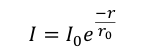Brightness exponential formula

Here $I_0$ is a constant that varies from galaxy to galaxy, r is the radius from the center of the galaxy, and $r_0$ is a constant that roughly describes the size of the disk. $r_0$ is typically on the order of 104 light years. Since the surface brightness tells us how many stars there are, the surface density of matter at any particular radius from the center also has a fall off given by the same type of expression.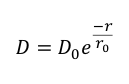Surface density formula

Here, $D$ is the surface density of luminous matter in $kg/m^2$ and $D_0$ is a constant. We can observe this decline of density in most galaxies at least out to 4 or 5 times $r_0$. You may be used to thinking about densities as volume densities, $kg/m^3$. However, since a spiral galaxy disk is very thin compared to its size across the disk, it can be thought of as a two dimensional object. Therefore the relevant quantity is a surface density. Measurements of the surface density of luminous matter, allow physicists to measure the amount and distribution of visible matter in a galaxy.

The previous discussion implies that if we look at the stars towards the edges of the galaxy, the amount of mass inside their orbit is almost the entire mass of the galaxy. Well outside the central bulge, the gravitational mass attracting stars is essentially a constant as we move out in radius $r$ from the galactic center (assuming all the matter is luminous). The gravitational force on those stars is therefore: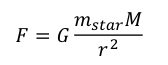Gravitational force equation

where $M$ is just the mass of the galaxy. These stars move in circular orbits around the center, so they must have a centripetal acceleration given by:Centripetal acceleration formula

By Newton’s second law, $F_{Net}=ma$, we know that the net force is just the gravitational force. We can solve for the velocity of a star as it orbits the center of the galaxy: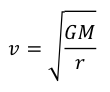Velocity of star orbit

The expected velocity of stars in a galaxy should therefore decrease by $r^{\frac{1}{2}}$ as we move to the outer edges of galaxies.

We can actually measure the velocity of stars as they orbit and compare them to predictions based off of these relationships. We do this in the following way. If you look at the sun during the day, it’s vaguely yellowish. However, the sun actually emits many different colors of light. If you place a prism in direct sunlight, the light from the sun will split into a rainbow of colors with some dark gaps, as shown in the figure below.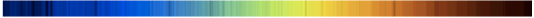Spectrum of sunlight

Spectrum of sunlight

The dark lines occur because the outer layers of gas in the sun actually absorb certain colors of light, preventing them from reaching our instruments and eyes.. These spectral lines are characteristic of stars, not just our own sun. We know very accurately which colors should be absorbed by the gases in a star. By measuring the spectrum of a star and comparing the absorption lines to where they should be, we can measure the velocities of stars.

Imagine standing in front of a fire engine traveling towards you. The siren sound you hear is higher pitched than if the fire truck was standing still. As the fire truck drives by, the siren pitch decreases. Nothing changed about the actual siren, all that changed was that the truck went from moving towards you to away from you. This effect is called the Doppler effect, and it works for light as well as sound.

A star moving towards you has the dark lines shifted towards the blue end of the spectrum, just as the siren of a fire truck racing toward you is shifted to higher pitch. Stars moving away from us have dark lines shifted towards the red end of the spectrum, just as the siren of a fire truck is shifted to a lower pitch when it has passed you and is traveling away. By measuring where the lines occur relative to where they should occur, we can determine the velocity of stars.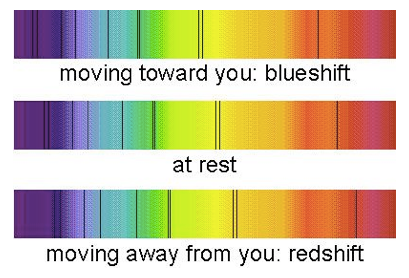Redshift blueshift

Redshift vs. Blueshift. Credit:(astronomynotes)

Using this technique we can observe the actual velocities of stars orbiting galaxies and compare them with what Newton’s 2nd Law and the Law of Universal Gravitation predict. These predicted velocities are based off of accounting for all of the mass in a galaxy that we can see (through measuring the surface density of luminous matter). Below is a graph of the predicted vs. expected, rotational velocities as a function of radius (or “rotation curve”) for a typical spiral galaxy. The radial distance is in kilo-parsecs, where 1 parsec is 3.26 light years.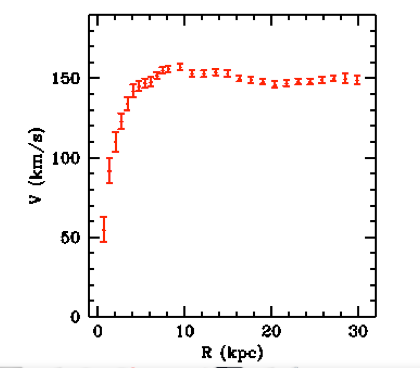Rotation curve of a typical spiral galaxy Rotation curve of a typical spiral galaxy NGC3198, from Begeman, 1989

Does this look like it falls off as a function of $r$? No, not really. The velocity for large radii is essentially constant instead. If you plot the predicted vs. observed rotation curve on the same graph for just about any spiral galaxy you get a picture like this: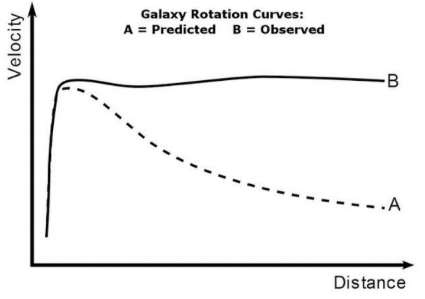Predicted vs. observed galactic rotation curve Predicted vs. observed galactic rotation curve, from Begemen 1991

These observations do not look like the predictions in the least.

The peaks or rises at the beginning of the curves above are due to the large numbers of stars in the bulge. Near the central bulge there are so many stars that the gravitational mass attracting a star at radius r away from the galactic center changes significantly as the radius changes. Once we get outside the bulge the predicted velocity vs. distance curve takes the $r^\frac{1}{2}$ dependence.

The observed versus predicted curves look nothing alike and the error bars (as you can see in the Galactic rotation curve for NGC3198, for example) are quite small. Therefore there is a big discrepancy. Since the observations are repeatable and straightforward for many spiral galaxies, and Newton’s laws are very fundamental and well understood, something in our underlying assumptions must be wrong!

The most accepted hypothesis that corrects the predicted velocity graph to match the observed graph, is that most of the matter in a galaxy is actually not luminous matter, but is instead dark matter, or matter that doesn’t glow. The assumption that all matter in a a galaxy is luminous and visible, is likely false.

Additionally, the distribution of dark matter must not follow the same distribution as the luminous matter. The first part: that the matter must be present but dark, stems from the combination of the rotation curves and surface brightness graphs. Since the rotation curves are different, some extra matter must be present, but since we can account for all luminous matter in the surface brightness observations, it can’t give off light. The distribution of dark matter must then be chosen to match the flat rotation curve at the edges of the galaxy. Dark matter is usually modeled as a halo around the galaxy that does not arrange itself into a disk, but is more similar in shape to a sphere.

The above result is one of the primary reasons physicists believe in dark matter – you can match observations by adding dark matter to your galaxy. It was also one of the first. Note that constructing the dark matter to have this density profile is still an area of active research. However, the theory that is there, most physicists consider to be firmly established.

There are other signals of dark matter. We see light from distant galaxies bend due to gravity around regions of space where there doesn’t appear to be any luminous matter. We see possible signals of dark matter in the radiation we receive from our galaxy. Finally, the cosmological evolution of our universe also provides evidence for dark matter. There is much ongoing research to investigate these signals.

Bibliography

1. Rotation of the Andromeda Nebula from a Spectroscopic Survey of Emission Regions. Rubin, V and Ford, K. 1970, Astrophysical Journal.

2. H I rotation curves of spiral galaxies. I - NGC 3198. Begeman, K. 1989, Astronomy and Astrophysics, Vol. 223, pp. 47- 60.

3. Extended rotation curves of spiral galaxies - Dark haloes and modified dynamics. Begemen, K Broeils AH, Sanders RH. 1991, Monthly Notices of the Royal Astronomical Society, vol. 249, April 1,p. 523-537.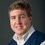Note by David Mattingly
7 years, 4 months ago

This discussion board is a place to discuss our Daily Challenges and the math and science related to those challenges. Explanations are more than just a solution — they should explain the steps and thinking strategies that you used to obtain the solution. Comments should further the discussion of math and science.

When posting on Brilliant:

• Use the emojis to react to an explanation, whether you're congratulating a job well done , or just really confused .
• Ask specific questions about the challenge or the steps in somebody's explanation. Well-posed questions can add a lot to the discussion, but posting "I don't understand!" doesn't help anyone.
• Try to contribute something new to the discussion, whether it is an extension, generalization or other idea related to the challenge.

MarkdownAppears as
*italics* or _italics_ italics
**bold** or __bold__ bold
- bulleted- list
• bulleted
• list
1. numbered2. list
1. numbered
2. list
Note: you must add a full line of space before and after lists for them to show up correctly
paragraph 1paragraph 2

paragraph 1

paragraph 2

[example link](https://brilliant.org)example link
> This is a quote
This is a quote
    # I indented these lines
# 4 spaces, and now they show
# up as a code block.

print "hello world"
# I indented these lines
# 4 spaces, and now they show
# up as a code block.

print "hello world"
MathAppears as
Remember to wrap math in $$ ... $$ or $ ... $ to ensure proper formatting.
2 \times 3 $2 \times 3$
2^{34} $2^{34}$
a_{i-1} $a_{i-1}$
\frac{2}{3} $\frac{2}{3}$
\sqrt{2} $\sqrt{2}$
\sum_{i=1}^3 $\sum_{i=1}^3$
\sin \theta $\sin \theta$
\boxed{123} $\boxed{123}$

Sort by:

I really like such stuff.

- 7 years, 2 months ago

liked it..

- 6 years, 6 months ago

Wow.... Great note! It really sheds light on this interesting topic.

- 5 years, 3 months ago

@David Mattingly I'd like to read your Wiki on pulsars.(It deals with conservation of Angular momentum.)

- 5 years, 2 months ago

@David Mattingly : Hi. I was reading an article some time ago, that stated that the increasing rate of the expansion of the universe is due to the presence of Dark Energy and Dark Matter, that try to cancel out the effect of gravity, that should slow this rate.

If that's the case, and the Dark stuff repels, then how do we account for the deviation from the observed graph. For the predicted graph to match the observed graph, as we move outwards, some extra mass must be there that cancels the effect of increasing radius. How can it be Dark Matter, if it exhibits repulsion, instead of attraction?

- 5 years, 6 months ago

Dark Matter doesn't exhibit repulsion, it's rather the Dark Energy that is the cause of expansion of our universe. The last paragraph of the above article also states that dark matter may be responsible for bending of light around non luminous regions of space, thus making the fact clear that dark matter attracts stuff. Hence, Dark Matter may be the solution to the deviation from observed graph

- 5 years, 6 months ago

I am not saying that Dark matter isn't the solution to the above graph, and yes, I did read that dark matter contributes to bending light, but so does ordinary matter! Gravitational lensing by black holes, can be explained with the help of ordinary matter.

Now, what my actual question was, that how come the matter and the energy, (which should follow the same properties) go against each other. If you consider Dark matter to be causing attraction, then how is Dark energy, which is the energy, due to dark matter, causing repulsion?

- 5 years, 6 months ago

Dark Energy isn't there due to Dark Matter, we don't know know anything about them both. Dark Energy is theorised to be a property of Empty Space on the basis of Cosmological Constant given by Einstein. Based on a theoretical model created keeping cosmological observations in mind the universe, scientists came up with the composition of the universe as 68% Dark Energy, 27% Dark Matter and 5% normal Matter (factual). So it's not surprising for them to show different behaviour, as they're not related to each other.

- 5 years, 6 months ago

What I meant by 'due to dark matter', actually referred to 'following the same properties'. And plus how can an energy just be created from out of nothing, i.e. Empty space?

- 5 years, 6 months ago